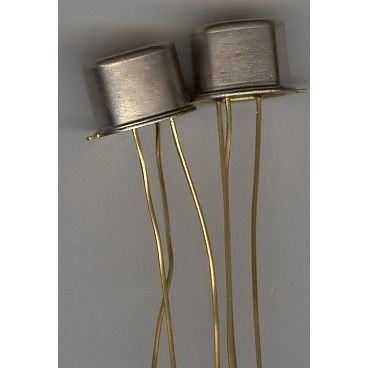•# TRANSISTOR 2N445 ELECTRONIC PARTS AVIONCS

\$9.00 \$4.00
Free Shipping!

TRANSISTOR

Part Number = 2N445
Manufacturer Name = Various
Description = Ge NPN Lo-Pwr BJT
V(BR)CEO (V) = 12
V(BR)CBO (V) = 15
Absolute Max. Power Diss. (W) = 100m
I(CBO) Max. (A) = 2.0u
@V(CBO) (V) (Test Condition) = 10
h(fe) Min. SS Current gain. = 35
@I(C) (A) (Test Condition) = 1.0m
@V(CE) (V) (Test Condition) = 5.0
@Freq. (Hz) (Test Condition) = 1.0k
f(T) Min. (Hz) Transition Freq = 2.0M
@I(C) (A) (Test Condition) = 1.0m
@V(CE) (V) (Test Condition) = 5.0
C(obo) (Max) (F) = 13p
@V(CB) (V) (Test Condition) = 5.0
@Freq. (Hz) (Test Condition) = 1.0M
Package = TO-5
Military = N

AIRCRAFT

AVIONICS

THIS SALE IS FOR 1 EACH

NEW SURPLUS

NOTE:

ALL ELECTRONICS ARE UNTESTED

SOLD AS IS